• 初始英文翻译
2022-05-31 08:47:07java
更多相关内容
• 易语言翻译英文复读机源码,翻译英文复读机,初始化,取网页文档对象,取IES窗口句柄,取网页属性,谷哥翻译,取中间文本,URL编码_utf8,Ansi转Utf8,Unicode转Utf8,Ansi转Unicode,喇叭朗读,GetClassName,...
• 思科初始化的翻译，基本配置的最初始化的英文进行翻译
• 在看英文资料时，对理解比较模糊的句子，希望看看别人是怎么理解的。最简单的方式就是用工具在线翻译一下。为了挑选一个合适的在线翻译，我进行了简单的比较。   英文原文，是一段技术文档： Objects are ...

在看英文资料时，对理解比较模糊的句子，希望看看别人是怎么理解的。最简单的方式就是用工具在线翻译一下。为了挑选一个合适的在线翻译，我进行了简单的比较。

英文原文，是一段技术文档：

Objects are structures allocated on the heap. Special rules apply to the use of objects to ensure they are properly garbage-collected.

Objects are never allocated statically or on the stack; they must be accessed through special macros and functions only.

(Type objects are exceptions to the first rule; the standard types are represented by statically initialized type objects,

although work on type/class unification for Python 2.2 made it possible to have heap-allocated type objects too).

几款免费在线翻译结果如下：

注：前面是个人主观评分，后面是产品名和网址。黄色字表示翻译出彩的(翻译出彩的)，红色划线字表示翻译严重错误的(翻译有语病的)。

对象是在堆上分配的结构。 特殊规则适用于使用对象确保它们被正确地垃圾收集。

永远不会静态地或在堆栈上分配对象; 他们一定是仅通过特殊宏和函数访问。

（类型对象是第一条规则的例外情况; 标准类型由表示静态初始化的类型对象，虽然在类型/类统一上工作对于Python 2.2，也可以使用堆分配的类型对象。

--- 88分 bing https://cn.bing.com/translator/

对象是在堆上分配的结构。 特殊规则适用于使用对象确保它们被正确地垃圾收集。

对象从不静态或在堆栈上分配;他们必须仅通过特殊的宏和函数访问。

(类型对象是第一条规则的例外情况;标准类型由静态初始化的类型对象, 虽然在类型/类统一上工作对于 Python 2.2, 也可以拥有堆分配的类型对象。

--- 90分 sogou fanyi.sogou.com

对象是在堆上分配的结构。特殊规则适用于使用对象确保它们被正确地垃圾收集。

对象从不静态分配或在堆栈上分配；他们一定是仅通过特殊宏和函数访问。

(类型对象是第一条规则的例外情况；标准类型由表示静态初始化的类型对象，尽管工作在类型/类统一上对于Python 2.2，也可以有堆分配的类型对象)

--- 80分 baidu

对象是堆上分配的结构。特殊规则适用于对象的使用确保它们被正确地垃圾收集。

对象永远不会静态分配或堆栈；它们必须是仅通过特殊宏和函数访问。

（类型对象是第一个规则的例外；标准类型由静态初始化类型对象，虽然在类型/类统一方面工作对于Python 2.2，也可以使用堆分配的类型对象。

--- 70分 youdao http://fanyi.youdao.com/

对象是在堆上分配的结构。特别规则适用于使用对象以确保正确地收集垃圾

对象从不静态或堆栈上分配;他们必须仅通过特殊宏和函数访问。

(类型对象第一项规则的例外情况;标准类型表示为静态初始化类型对象不过要处理类型/类的统一对于Python 2.2，也可以拥有堆分配的类型对象)

结论：

对这个测试例句，sogou翻译是最好的，基本没有错误，标点符号也处理的很好，而且还语句比较通顺。

baidu 和 youdao 有语句明显有语病，容易导致误解，还需要继续改进。

有比较专业的测评，也是sogou在线翻译评分最高，看来sogou的确有实力。

展开全文• 因为我英文不好，对接口、函数、结构体起名字的时候特别为难，因此我使用Python写了一个工具 在输入框输入对应的中文，就生成Golang语言对应的接口、函数或接口体的写法，如下演示： 看懂代码之后，就可以修改为...

因为我英文不好，对接口、函数、结构体起名字的时候特别为难，因此我使用Python写了一个工具
在输入框输入中文，就可以生成Golang语言对应的接口、函数或接口体的写法；也可以切换到英文翻译。如下演示：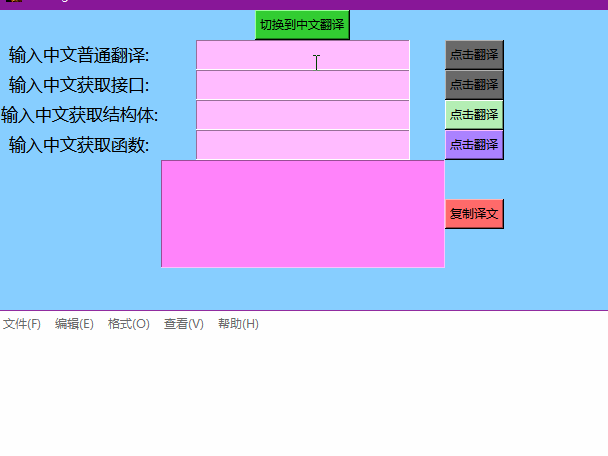看懂代码之后，就可以修改为其它语言对应的写法。这里使用到百度的翻译API，因此自己要替换掉下面的两个key值就直接可以用了。
Baidufanyi类用于中英文翻译；
Initface类用于创建窗口，之后调用第一个Frame，这里为了能切换不同页面，使用两个Frame作为两个页面
OneFace类是第一个页面，init函数用于初始化页面，faceUI方法用于显示控件和逻辑控制，并使用了grid布局，oneBack方法用于销毁本页面并切换到另一个页面
TwoFace类和第一个页面用法一致，页面使用了pack布局

# 翻译.py
import tkinter
import requests
import hashlib
import pyperclip
import time

class Baidufanyi:
def __init__(self, appid="百度API的应用ID", key="百度API的key"):
self.appid = appid
self.key = key
self.salt = "1435660288"

def getEnglish(self, srcdata):
q = srcdata
froms = "&from=zh"
tos = "&to=en"
sign = self.appid+q+self.salt+self.key
sign = "&sign="+hashlib.md5(sign.encode(encoding='utf-8')).hexdigest()
url = "http://api.fanyi.baidu.com/api/trans/vip/translate?"+"q="+q + \
froms+tos+"&appid="+self.appid+"&salt="+self.salt+sign
result = requests.get(url)
en_resu = eval(result.text)
return en_resu["trans_result"]["dst"]

def getChina(self, srcdata):
q = srcdata
froms = "&from=en"
tos = "&to=zh"
sign = self.appid+q+self.salt+self.key
sign = "&sign="+hashlib.md5(sign.encode(encoding='utf-8')).hexdigest()
url = "http://api.fanyi.baidu.com/api/trans/vip/translate?"+"q="+q + \
froms+tos+"&appid="+self.appid+"&salt="+self.salt+sign
result = requests.get(url)
en_resu = eval(result.text)
return en_resu["trans_result"]["dst"]

fanyi = Baidufanyi()

class Initface:
def __init__(self, windows):
self.windows = windows
self.windows.title("Golang翻译")
self.windows.iconbitmap('D:/Python代码/Python的py转exe/mingyu.ico')
self.windows.geometry('610x300')  # 设置窗口大小
self.windows.configure(background='#87CEFF')
OneFace(self.windows)  # 默认打开第一个页面

# 第一个页面
class OneFace:
def __init__(self, oneWindows):
self.oneWindows = oneWindows
self.oneface = tkinter.Frame(self.oneWindows)
self.oneface.configure(background='#87CEFF')
self.oneface.grid()
self.faceUI()

# 使用了grid布局，显示控件，里面的函数用于逻辑处理
def faceUI(self):
# 获取翻译结果并写入到大文本框
def getEn():
text.delete("0.0", "end")  # 删除大文本框的内容
srcdata = getTextInput(text_0).replace(
'\n', '').replace(' ', '')
if srcdata == "":
return
data = fanyi.getEnglish(srcdata)
text.insert("0.0", data)

# 获取翻译结果并写入到大文本框
def getInterface():
text.delete("0.0", "end")  # 删除大文本框的内容
srcdata = getTextInput(text_1).replace(
'\n', '').replace(' ', '')
if srcdata == "":
return
data = fanyi.getEnglish(srcdata).replace(
' ', '').replace('?', '').replace('\'', '')
data = "// " + srcdata + "接口\ntype " + \
data + "er" + " interface{\n\t\n}"
text.insert("0.0", data)

# 获取翻译结果并写入到大文本框

def getStruct():
text.delete("0.0", "end")  # 删除大文本框的内容
srcdata = getTextInput(text_2).replace(
'\n', '').replace(' ', '')
if srcdata == "":
return
data = fanyi.getEnglish(srcdata).replace(
' ', '').replace('\'', '').replace('?', '')
data = "// " + srcdata + "结构体\ntype " + \
data.capitalize() + " struct{\n\t\n}"
text.insert("0.0", data)

# 获取翻译结果并写入到大文本框

def getFunc():
text.delete("0.0", "end")  # 删除大文本框的内容
srcdata = getTextInput(text_3).replace(
'\n', '').replace(' ', '')
if srcdata == "":
return
data = fanyi.getEnglish(srcdata).replace(
' ', '').replace('?', '').replace('\'', '')
data = "// " + srcdata + "函数\nfunc " + \
data.capitalize() + "(  ) (err error) " + "{\n\t\n}"
text.insert("0.0", data)

def CopyData():
pyperclip.copy(text.get("0.0", "end"))
nowtime = time.strftime("%Y-%m-%d %H:%M:%S", time.localtime())
tishistr.set('已复制\n' + nowtime)

# 根据多行文本的变量名获取里面的值，textname：多行文本的变量名
def getTextInput(textname):
return textname.get("1.0", "end")

oneBtn_1 = tkinter.Button(
self.oneface, text="切换到中文翻译", bg="#32CD32", command=self.oneBack)
oneBtn_1.grid(row="0", column="2")

china0 = tkinter.Label(
self.oneface, text="输入中文普通翻译:", bg='#87CEFF', font=("微软雅黑", 13))
china0.grid(row="1", column="1")
text_0 = tkinter.Text(self.oneface, width="30",
height="2", bg='#FFBBFF')
text_0.grid(row="1", column="2")
btn_0 = tkinter.Button(
self.oneface, text="点击翻译", command=getEn, bg="#696969")
btn_0.grid(row="1", column="3")

china1 = tkinter.Label(
self.oneface, text="输入中文获取接口:", bg='#87CEFF', font=("微软雅黑", 13))
china1.grid(row="2", column="1")
text_1 = tkinter.Text(self.oneface, width="30",
height="2", bg='#FFBBFF')
text_1.grid(row="2", column="2")
btn_1 = tkinter.Button(
self.oneface, text="点击翻译", command=getInterface, bg="#696969")
btn_1.grid(row="2", column="3")

china2 = tkinter.Label(
self.oneface, text="输入中文获取结构体:", bg='#87CEFF', font=("微软雅黑", 13))
china2.grid(row="3", column="1")
text_2 = tkinter.Text(self.oneface, width="30",
height="2", bg='#FFBBFF')
text_2.grid(row="3", column="2")
btn_2 = tkinter.Button(
self.oneface, text="点击翻译", command=getStruct, bg="#B4EEB4")
btn_2.grid(row="3", column="3")

china3 = tkinter.Label(
self.oneface, text="输入中文获取函数:", bg='#87CEFF', font=("微软雅黑", 13))
china3.grid(row="4", column="1")
text_3 = tkinter.Text(self.oneface, width="30",
height="2", bg='#FFBBFF')
text_3.grid(row="4", column="2")
btn_3 = tkinter.Button(
self.oneface, text="点击翻译", command=getFunc, bg="#AB82FF")
btn_3.grid(row="4", column="3")

# 多行文本输入框
tishistr = tkinter.StringVar()
tishi = tkinter.Label(
self.oneface, textvariable=tishistr, bg='#87CEFF', font=("微软雅黑", 10))
tishi.grid(row="5", column="1")
text = tkinter.Text(self.oneface, width="40",
height=8, bg='#FF83FA')
text.grid(row="5", column="2")
btn_4 = tkinter.Button(
self.oneface, text="复制译文", command=CopyData, bg="#FF6A6A")
btn_4.grid(row="5", column="3")

def oneBack(self):
self.oneface.destroy()
TwoFace(self.oneWindows)

# 第二个页面
class TwoFace():
def __init__(self, twoWindows):
self.twoWindows = twoWindows
self.twoface = tkinter.Frame(self.twoWindows)
self.twoface.config(bg='#87CEFF')
self.twoface.pack()
self.faceUI()

# 使用了pack布局，显示控件
def faceUI(self):
# 根据多行文本的变量名获取里面的值，textname：多行文本的变量名
def getTextInput(textname):
return textname.get("1.0", "end")

def getCh():
twoText_2.delete("0.0", "end")  # 删除大文本框的内容
srcdata = getTextInput(twoText_0).replace(
'\n', '').replace(' ', '')
if srcdata == "":
return
data = fanyi.getChina(srcdata)
twoText_2.insert("0.0", data)

twoBtn_1 = tkinter.Button(
self.twoface, text="切换到英文翻译", bg="#32CD32", command=self.twoBack)
twoBtn_1.pack()
twoText_0 = tkinter.Text(
self.twoface, width="50", height="8", bg='#FF83FA')
twoText_0.pack()
twoBtn_0 = tkinter.Button(
self.twoface, text="点击翻译", bg="#7B68EE", command=getCh)
twoBtn_0.pack()
twoText_2 = tkinter.Text(
self.twoface, width="70", height="8", bg='#FF83FA')
twoText_2.pack()

# 切换到其他页面
def twoBack(self):
self.twoface.destroy()  # 销毁页面
OneFace(self.twoWindows)  # 切换到另一个页面

if __name__ == '__main__':
windows = tkinter.Tk()
Initface(windows)
windows.mainloop()



然后使用命令打包

 pyinstaller -F -w -i D:\Python代码\Python的py转exe\mingyu.ico D:\Python代码\Python的py转exe\翻译.py


得到一个exe的文件展开全文python tkinter
• ## 基于keras的seq2seq中英文翻译实现

万次阅读 多人点赞 2018-07-13 09:58:30
1. seq2seq概述 ...2. 中英文翻译实战 2.1 数据处理 2.2 encoder-decoder模型搭建 2.2.1 用于训练的模型 2.2.2 用于推理的模型 2.3 模型评价 3 致谢 本文详细代码见 ...

# 1. seq2seq概述

## 1.1 seq2seq简介

seq2seq，全称Sequence to sequence，是RNN结构的一个变形，来自于Cho 在 2014 年提出的 Encoder–Decoder 结构，https://arxiv.org/pdf/1406.1078.pdf
传统的RNN输入和输出长度要一致，而seq2seq在RNN的基础上进行改进，实现了变长序列的输入和输出，广泛的应用在了机器翻译、对话系统、文本摘要等领域。
【seq2seq同时可作为一种特征提取器来提取特征，可参考论文A method for estimating process maliciousness with Seq2Seq model

seq2seq与Encoder-Decoder:
Encoder-decoder是模拟人类认知的一个过程, encoder记忆和理解信息，并提炼信息通常会形成一个低维向量，decoder回忆与运用这些信息，再将加工后的信息输出来。
Encoder和Decoder部分可以是任意的文字，语音，图像，视频数据，模型可以采用CNN，RNN，Bi-RNN、LSTM、GRU等等。当encoder和decoder处理的都是序列时称为seq2seq。

## 1.2 seq2seq结构

seq2seq由encoder和decoder组成。
encoder负责将输入序列压缩成指定长度的向量，这个向量就可以看成是这个序列的语义，这个过程称为编码，如下图，获取语义向量最简单的方式就是直接将最后一个输入的隐状态作为语义向量C。也可以对最后一个隐含状态做一个变换得到语义向量，还可以将输入序列的所有隐含状态做一个变换得到语义变量。
decoder则负责根据语义向量生成指定的序列，这个过程也称为解码。如下图，这是一种简单的seq2seq，最简单的方式是将encoder得到的语义变量作为初始状态输入到decoder的RNN中，得到输出序列。可以看到上一时刻的输出会作为当前时刻的输入，而且其中语义向量C只作为初始状态参与运算，后面的运算都与语义向量C无关。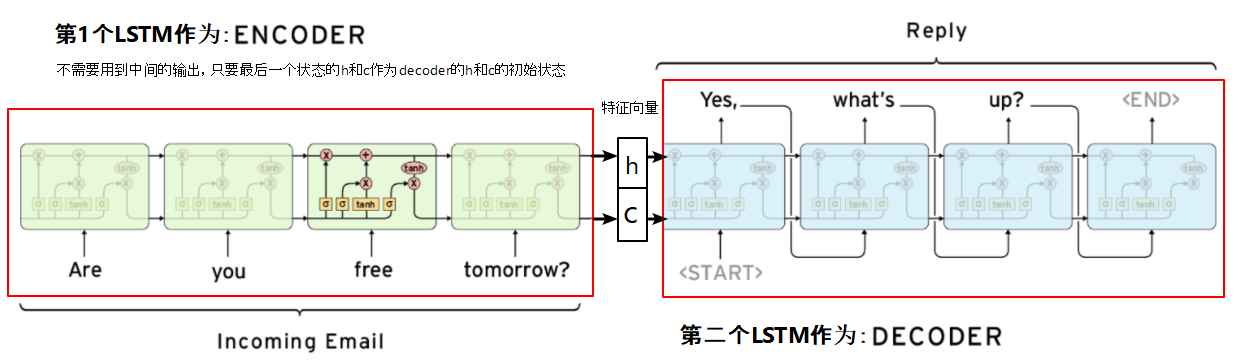# 2. 中英文翻译实战

本文使用中英文翻译数据集，来实现字符级的seq2seq模型的训练。
该文件来自于http://www.manythings.org/anki/，包含了20133条中英文翻译。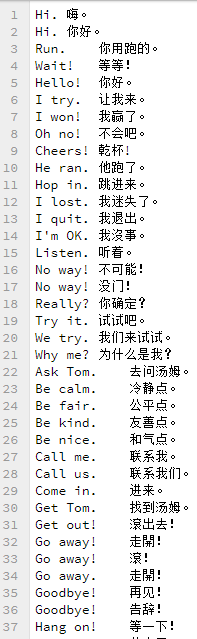## 2.1 数据处理

首先要将数据处理成Keras中模型接受的三维向量。这里需要处理3个向量，分别是encoder的输入encoder_input，decoder的输入和输出decoder_inputdecoder_output

 #读取cmn-eng.txt文件
data_path = 'data/cmn.txt'
df.columns=['inputs','targets']
#讲每句中文句首加上'\t'作为起始标志，句末加上'\n'作为终止标志
df['targets'] = df['targets'].apply(lambda x: '\t'+x+'\n')

input_texts = df.inputs.values.tolist()#英文句子列表
target_texts = df.targets.values.tolist()#中文句子列表

#确定中英文各自包含的字符。df.unique()直接取sum可将unique数组中的各个句子拼接成一个长句子
input_characters = sorted(list(set(df.inputs.unique().sum())))
target_characters = sorted(list(set(df.targets.unique().sum())))

每条句子经过对字母转换成one-hot编码后，生成了LSTM需要的三维输入[n_samples, timestamp, one-hot feature]

#encoder输入、decoder输入输出初始化为三维向量
encoder_input = np.zeros((NUM_SAMPLES,INUPT_LENGTH,INPUT_FEATURE_LENGTH))
decoder_input = np.zeros((NUM_SAMPLES,OUTPUT_LENGTH,OUTPUT_FEATURE_LENGTH))
decoder_output = np.zeros((NUM_SAMPLES,OUTPUT_LENGTH,OUTPUT_FEATURE_LENGTH))

其中：

• NUM_SAMPLES，样本条数，这里是输入的句子条数
• INPUT_LENGTH，输入数据的时刻t的长度，这里为最长的英文句子长度
• OUTPUT_LENGTH，输出数据的时刻t的长度，这里为最长的中文句子长度
• INPUT_FEATURE_LENGTH，每个时刻进入encoder的lstm单元的数据 xt x t $x_t$的维度，这里为英文中出现的字符数
• OUTPUT_FEATURE_LENGTH，每个时刻进入decoder的lstm单元的数据 xt x t $x_t$的维度，这里为中文中出现的字符数

对句子进行字符级one-hot编码，将输入输出数据向量化：


#encoder的输入向量one-hot
for seq_index,seq in enumerate(input_texts):
for char_index, char in enumerate(seq):
encoder_input[seq_index,char_index,input_dict[char]] = 1
#decoder的输入输出向量one-hot，训练模型时decoder的输入要比输出晚一个时间步，这样才能对输出监督
for seq_index,seq in enumerate(target_texts):
for char_index,char in enumerate(seq):
decoder_input[seq_index,char_index,target_dict[char]] = 1.0
if char_index > 0:
decoder_output[seq_index,char_index-1,target_dict[char]] = 1.0

这里，查看获得的三个向量如下：

In:  ''.join([input_dict_reverse[np.argmax(i)] for i in encoder_input if max(i) !=0])
Out: 'Hi.'

In:  ''.join([input_dict_reverse[np.argmax(i)] for i in decoder_output if max(i) !=0])
Out: '嗨。\n'

In:  ''.join([input_dict_reverse[np.argmax(i)] for i in decoder_input if max(i) !=0])
Out: '\t嗨。\n'

其中input_dicttarget_dict为中英文字符与其索引的对应词典；input_dict_reversetarget_dict_reverse与之相反，索引为键字符为值：

input_dict = {char:index for index,char in enumerate(input_characters)}
input_dict_reverse = {index:char for index,char in enumerate(input_characters)}
target_dict = {char:index for index,char in enumerate(target_characters)}
target_dict_reverse = {index:char for index,char in enumerate(target_characters)}

## 2.2 encoder-decoder模型搭建

我们预测过程分为训练阶段和推理阶段，模型也分为训练模型和推断模型，这两个模型encoder之间deocder之间权重共享。
为什么要这么划分的？我们仔细考虑训练过程，会发现训练阶段和预测阶段的差异。
在训练阶段，encoder的输入为time series数据，输出为最终的隐状态，decoder的输出应该是target序列。为了有监督的训练，decoder输入应该是比输入晚一个时间步，这样在预测时才能准确的将下一个时刻的数据预测出来。
在训练阶段，每一时刻decoder的输入包含了上一时刻单元的状态 ht1 h t − 1 $h_{t-1}$ ct1 c t − 1 $c_{t-1}$，输出则包含了本时刻的状态 h h $h$$c$$c$以及经过全连接层之后的输出数据。
训练时的流程：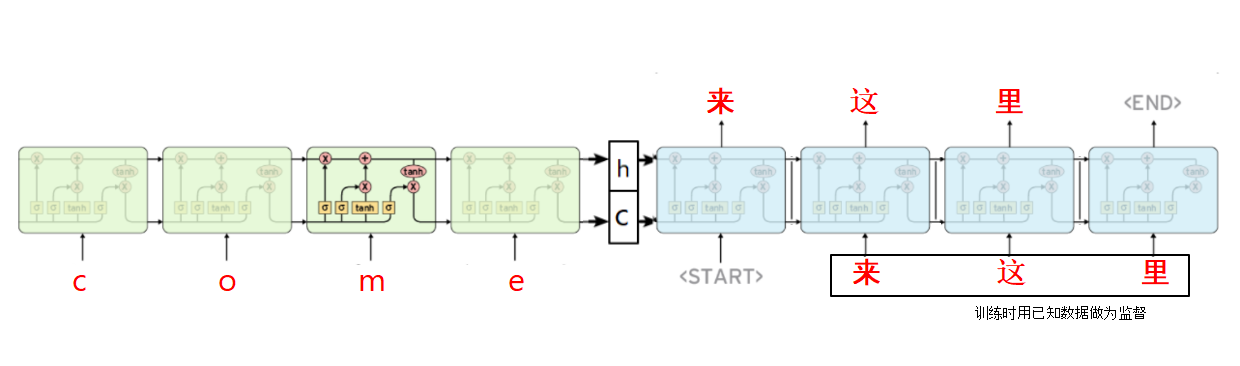预测时的流程：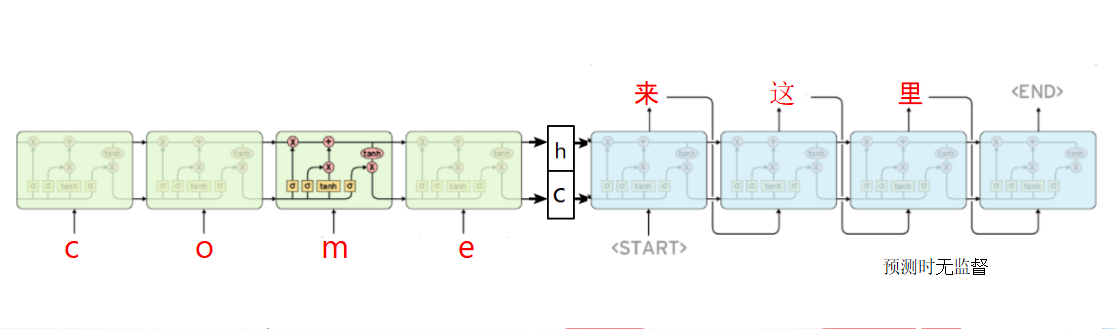### 2.2.1 用于训练的模型


#encoder
encoder_input = Input(shape = (None, n_input))
#encoder输入维度n_input为每个时间步的输入xt的维度，这里是用来one-hot的英文字符数
encoder = LSTM(n_units, return_state=True)
#n_units为LSTM单元中每个门的神经元的个数，return_state设为True时才会返回最后时刻的状态h,c(详见参考文献5,6)
_,encoder_h,encoder_c = encoder(encoder_input)
encoder_state = [encoder_h,encoder_c]
#保留下来encoder的末状态作为decoder的初始状态

#decoder
decoder_input = Input(shape = (None, n_output))
#decoder的输入维度为中文字符数
decoder = LSTM(n_units,return_sequences=True, return_state=True)
#训练模型时需要decoder的输出序列来与结果对比优化，故return_sequences也要设为True
decoder_output, _, _ = decoder(decoder_input,initial_state=encoder_state)
#在训练阶段只需要用到decoder的输出序列，不需要用最终状态h.c
decoder_dense = Dense(n_output,activation='softmax')
decoder_output = decoder_dense(decoder_output)
#输出序列经过全连接层得到结果

#生成的训练模型
model = Model([encoder_input,decoder_input],decoder_output)
#第一个参数为训练模型的输入，包含了encoder和decoder的输入，第二个参数为模型的输出，包含了decoder的输出


训练模型：
其中英文字符（含数字和符号）共有73个，中文中字符（含数字和符号）共有2623个。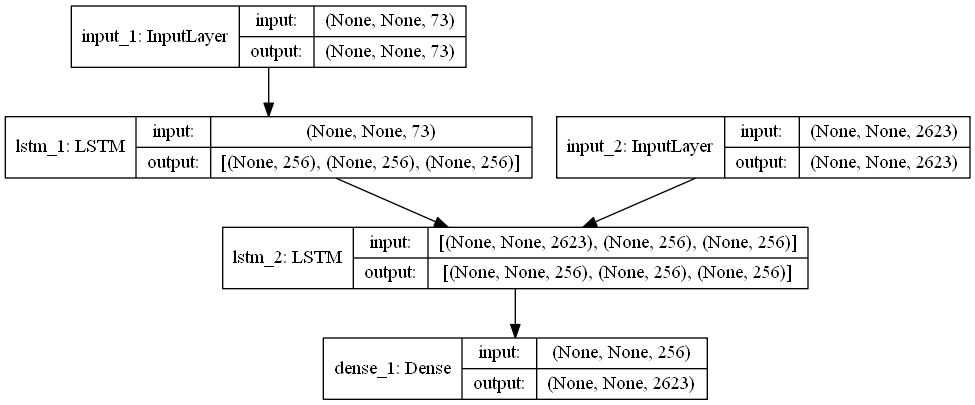### 2.2.2 用于推理的模型

 #推断模型-encoder，预测时对序列predict，生成的state给decoder
encoder_infer = Model(encoder_input,encoder_state)

encoder推断模型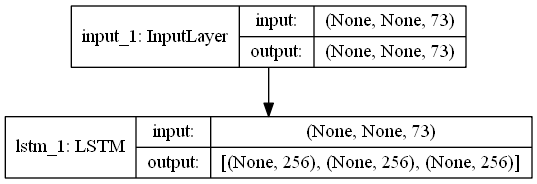#推断模型-decoder
decoder_state_input_h = Input(shape=(n_units,))
decoder_state_input_c = Input(shape=(n_units,))
decoder_state_input = [decoder_state_input_h, decoder_state_input_c] #上个时刻的状态h,c

decoder_infer_output, decoder_infer_state_h, decoder_infer_state_c = decoder(decoder_input,initial_state=decoder_state_input)
decoder_infer_state = [decoder_infer_state_h, decoder_infer_state_c]#当前时刻得到的状态
decoder_infer_output = decoder_dense(decoder_infer_output)#当前时刻的输出
decoder_infer = Model([decoder_input]+decoder_state_input,[decoder_infer_output]+decoder_infer_state)


decoder推断模型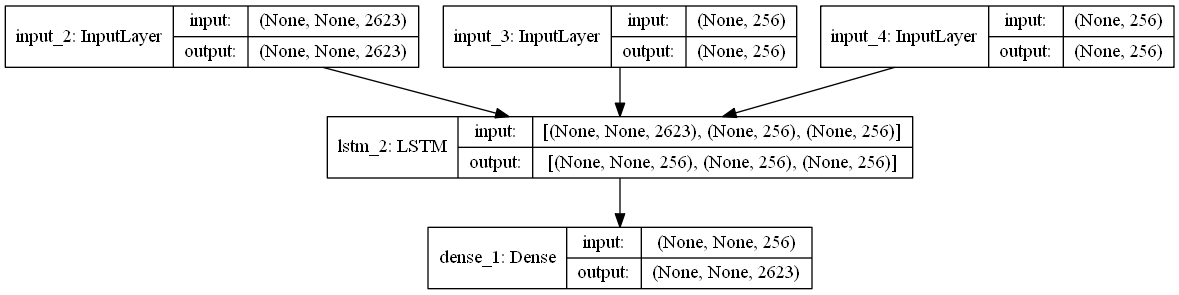把模型创建整理成一个函数：

def create_model(n_input,n_output,n_units):
#训练阶段
encoder_input = Input(shape = (None, n_input))
encoder = LSTM(n_units, return_state=True)
_,encoder_h,encoder_c = encoder(encoder_input)
encoder_state = [encoder_h,encoder_c]

#decoder
decoder_input = Input(shape = (None, n_output))
decoder = LSTM(n_units,return_sequences=True, return_state=True)
decoder_output, _, _ = decoder(decoder_input,initial_state=encoder_state)
decoder_dense = Dense(n_output,activation='softmax')
decoder_output = decoder_dense(decoder_output)

#生成的训练模型
model = Model([encoder_input,decoder_input],decoder_output)

#推理阶段，用于预测过程
encoder_infer = Model(encoder_input,encoder_state)

decoder_state_input_h = Input(shape=(n_units,))
decoder_state_input_c = Input(shape=(n_units,))
decoder_state_input = [decoder_state_input_h, decoder_state_input_c]#上个时刻的状态h,c

decoder_infer_output, decoder_infer_state_h, decoder_infer_state_c = decoder(decoder_input,initial_state=decoder_state_input)
decoder_infer_state = [decoder_infer_state_h, decoder_infer_state_c]#当前时刻得到的状态
decoder_infer_output = decoder_dense(decoder_infer_output)#当前时刻的输出
decoder_infer = Model([decoder_input]+decoder_state_input,[decoder_infer_output]+decoder_infer_state)

return model, encoder_infer, decoder_infer

## 2.3 模型评价

def predict_chinese(source,encoder_inference, decoder_inference, n_steps, features):
#先通过推理encoder获得预测输入序列的隐状态
state = encoder_inference.predict(source)
#第一个字符'\t',为起始标志
predict_seq = np.zeros((1,1,features))
predict_seq[0,0,target_dict['\t']] = 1

output = ''
#开始对encoder获得的隐状态进行推理
#每次循环用上次预测的字符作为输入来预测下一次的字符，直到预测出了终止符
for i in range(n_steps):#n_steps为句子最大长度
#给decoder输入上一个时刻的h,c隐状态，以及上一次的预测字符predict_seq
yhat,h,c = decoder_inference.predict([predict_seq]+state)
#注意，这里的yhat为Dense之后输出的结果，因此与h不同
char_index = np.argmax(yhat[0,-1,:])
char = target_dict_reverse[char_index]
output += char
state = [h,c]#本次状态做为下一次的初始状态继续传递
predict_seq = np.zeros((1,1,features))
predict_seq[0,0,char_index] = 1
if char == '\n':#预测到了终止符则停下来
break
return output

对100个值进行预测：

for i in range(1000,1210):
test = encoder_input[i:i+1,:,:]#i:i+1保持数组是三维
out = predict_chinese(test,encoder_infer,decoder_infer,OUTPUT_LENGTH,OUTPUT_FEATURE_LENGTH)
print(input_texts[i])
print(out)

我们发现预测的都很准（废话，训练集能不准嘛！）
翻译结果：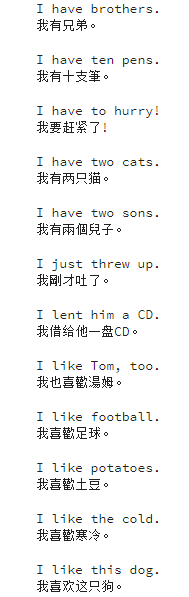# 3 致谢

本文参照了以下文章：
 keras/examples/lstm_seq2seq.py, https://github.com/keras-team/keras/blob/master/examples/lstm_seq2seq.py
 A ten-minute introduction to sequence-to-sequence learning in Keras, https://blog.keras.io/a-ten-minute-introduction-to-sequence-to-sequence-learning-in-keras.html
 How to Develop an Encoder-Decoder Model for Sequence-to-Sequence Prediction in Keras, https://machinelearningmastery.com/develop-encoder-decoder-model-sequence-sequence-prediction-keras/
 Keras-9 实现Seq2Seq, https://blog.csdn.net/weiwei9363/article/details/79464789
 理解LSTM在keras API中参数return_sequences和return_state, https://blog.csdn.net/u011327333/article/details/78501054
 Keras关于LSTM的units参数，还是不理解? https://blog.csdn.net/xiewenbo/article/details/79452843

展开全文seq2seq keras 机器翻译 LSTM
• Effective Modern C++英文版及中文翻译. 第一章 类型推导 条款1： 理解模板类型推导 条款2： 理解auto类型推导 条款3： 理解decltype 条款4： 知道如何查看类型推导 第二章 auto关键字 条款5： 优先使用auto而非显式...
• 英文切换-国际化1.先看效果2.核心代码(重新赋值刷新翻译文本)2.0 pro文件配置2.1 构造函数2.2 两个按钮点击事件3.翻译文件的生成与配置4. 参考链接 1.先看效果 ... // .h中的变量 这里初始化分配内存 uiQt国际化 Qt中英文切换
•机器翻译
• 该模型实现的是英文到中文的翻译，下图为了更好展示模型架构用的大佬的图： 整体由encoder和decoder两大部分组成，每部分都有一个LSTM网络，其中encoder输入原始的句子，decoder输入的是含有开始符号的翻译后的...lstm 机器翻译 机器学习
• 英文：big data，mega data 大数据，或称巨量资料，指的是需要新处理模式才能具有更强的决策力、洞察发现力和流程优化能力的海量、高增长率和多样化的信息资产。 二、大数据的4V： Volume（大量）、Velocity...
• 初始朗读速度,更新列表,英文翻译引擎,中文翻译引擎,读数据
• 在第一次使用局部静态对象前创建并初始化它，当程序结束时局部静态对象才被销毁。 （ 11）函数（function） 可调用的计算单元。它对程序的结构化至关重要。每个函数都包含返回类型、名字、（可能为空）形参列表...
• 学习C++ Primer 时对一些常用术语（包括对应英文）及其简单用法的总结。
• 最近一个项目上有要求完成app国际化 也就是如果系统语言是英文，那么你的app打开时就会自动读取string 中的字符串资源，自动完成匹配，以满足国际化需求，那么我们就按照步骤走，完成我们的app国际化道路。...
• 大陈心里惦记着明天的5节课如何才能上好，把Python编辑器前前后后翻了一下，在Help菜单里找到了“IDLE Help”(如图1所示)，英语不好的大陈大致看了一下，原来是Python的IDLE和Shell中的菜单说明，尝试了百度翻译之后...
• 编译错误类型以及其原因还有中文翻译 作为一名C++的学习者（任何语言都是一样的），学会读编译器发出的错误信息都是一件很重要的事情。在前辈们不断地犯错的过程中，渐渐都会形成自己的错误调试体系。这里将绝大...
• 【OpenCV】Mat的初始化和非初始化设值一、初始化设值例一例二二、非初始化设值例一例二...此为OpenCV的官方教程，英文版，浏览器有翻译功能的话可以翻译来看，整体大概还是看得懂的。 一、初始化设值 例一 直接进入...OpenCV Mat 初始化
• TMS320F28377xD技术参考手册中文翻译dsp，technical reference manual手册中文翻译
• 2.1前端项目初始化步骤 安装 Vue 脚手架 通过 Vue 脚手架创建项目 配置 Vue 路由 配置 Element-UI 组件库 配置 axios 库 初始化 git 远程仓库 (前端项目必须使用远程仓库代码管理) 将本地项目托管到GitHhub...
• msgstr "计算季节性初始值至少需要两个周期的数据" msgid "invalid length(x)" msgstr "length(x)不对" msgid "optimization difficulties: %s" msgstr "优化困难： %s " msgid "optimization failure" msgstr ...
• class [klɑ:s] 类 classpath [klɑ:s’pɑ:θ ] 类路径 public ['p ʌblik] 公共的,公用的 private ['praivit] 私有的,私人的 static ['stæ tik] 静的;静态的;静止的 void [vɔid] 空的,没有返回值的 ...java
• ## 英文题面翻译

千次阅读 2022-01-15 14:15:42
英文题面: http://noi.openjudge.cn/ch0201/755/ 翻译: 在一个由16个双面正方形拼成的一个4*4的矩形上玩翻转游戏。每个小正方形的一面是白色的，另一面是黑色的。它们都平放在上面，黑的或白的一面朝上。每一轮你...
• 今天准备翻译的网站是 socket . io 网址链接： https://socket.io/get-started/chat/ 首先是左侧sidebar部分翻译： Introduction - - 介绍，入门 The web framework - - web框架 Serving HTML - - 个人理解是服务器...
• 说明一个类的数据成员的初始值，在构造函数体执行之前首先用初始值列表中的值初始化数据成员。未经初始值列表初始化的成员将被默认初始化。 （11）转换构造函数（converting constructor） 可以用一个实参调用的...
• Please take moment to perform ...百度翻译： 请采取时刻来执行初始配置 perform： perform [per·form || pər’fɔrm /pə’fɔːm] v. 履行; 完成; 执行; 做; 演出, 演奏, 表演; 行动, 表现; 运转 initial：
• ######一个为日文/英文翻译服务的人们提供信息的网站 初始目标网页 报价页面 ######说明：用户可以查看翻译率的报价。 联系页面 ######说明：用户可以填写联系表格。 日语页面 ######说明：用户可以在英语和日语之间...
• ## SD卡初始化细节

千次阅读 2018-07-27 14:10:43
遇到了初始化中检测SD卡类型的问题,查看学习板带的源程序发现使用了CMD8命令进行判断SD卡的类型,在网上查了好久查不到有关SDV2.0中CMD8命令的详细描述,于是自己看了英文版的SDV2.0协议(2006),找出了CMD8命令的章节,...SD初始化...